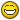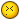# Reset one instance of a block and it's attributes to the defaults of that block## Recommended PostsHello,

Using the sync attributes (attsync), resets all the attributes properties of all occurrences of that block in the drawing to the defaults.

Anyone has a lisp that will reset all properties of one instance of a block to the default properties including attributes?

Only the picked instance or several occurrence picked should be reset by the lisp.

Thank you

##### Share on other sitesIs reinserting an option?

Do you want to retain the original X/Y/Z block scales and rotation?

Or you just want to reset the attribute values to their default?

##### Share on other sitesReinserting will ruin the values of the attributes.

I need to reset the only the properties to default.

Like the rotation, Height, visibility and so on.

Regards

##### Share on other sitesYes, I want to retain the original X/Y/Z block scales and rotation.

##### Share on other sitesHeres one reinserting approach:

```(defun C:test ( / SS spc i nm e1 o1 enx1 e2 o2 enx2 L blks )
(and
(princ "\nSelect Attributed blocks: ")
(setq SS (ssget "_:L" '((0 . "INSERT")(66 . 1))))
(setq spc (getvar 'ctab))
(repeat (setq i (sslength SS))
(and
(setq o1 (vlax-ename->vla-object (setq e1 (ssname SS (setq i (1- i))))))
(VanillaINSERT (vlax-get o1 'InsertionPoint) (setq nm (vlax-get o1 'EffectiveName)) spc)
(progn
(foreach x
(mapcar 'list
(vlax-invoke o1 'GetAttributes)
(vlax-invoke (setq o2 (vlax-ename->vla-object (setq e2 (entlast)))) 'GetAttributes)
)
(apply (function (lambda (a b) (vla-put-TextString b (vla-get-TextString a)))) x)
)
(mapcar 'set '(enx1 enx2) (mapcar 'entget (list e1 e2)))
(foreach x '(8 41 42 43 50)
(setq enx2 (subst (assoc x enx1) (assoc x enx2) enx2))
)
(entmod enx2)
(and
(eq (vla-get-IsDynamicBLock o1) :vlax-true)
(setq L (vlax-invoke o1 'GetDynamicBlockProperties))
(foreach x (mapcar 'list L (vlax-invoke o2 'GetDynamicBlockProperties))
(vl-catch-all-apply
(function
(lambda (a b)
(vla-put-Value b (vla-get-Value a))
); lambda
); function
x
)
); foreach
); and
(entdel e1) (setq blks (cons (if (not (member nm blks)) nm) blks)) T
); progn
); and
); repeat
(and (setq blks (vl-remove 'nil blks))
(setvar 'cmdecho 0)
(foreach x (vl-remove 'nil blks) (command "_.ATTSYNC" "_N" x) )
(setvar 'cmdecho 1)
)
); and
(princ)
); defun

; (VanillaINSERT (getpoint) "VLD_Tag" (getvar 'ctab))
(defun VanillaINSERT ( p nm spc / GetBlkAttDefProps atts r )
; '(87 114 105 116 116 101 110 32 66 121 32 71 114 114 114)
(setq GetBlkAttDefProps
(lambda ( bnm / e enx typ L )
(and
(setq e (tblobjname "BLOCK" bnm))
(= 2 (logand 2 (cdr (assoc 70 (setq enx (entget e))))))
(setq e (cdr (assoc -2 enx)))
(while (and e (setq enx (entget e)) (/= "SEQEND" (setq typ (cdr (assoc 0 enx)))))
(if (= "ATTDEF" typ) (setq L (cons (vl-remove-if-not (function (lambda (x) (member (car x) '(1 2 3 7 10 11 40)))) enx) L)))
(setq e (entnext e))
); while
); and
(reverse L)
); lambda
); setq GetBlkAttDefProps

(cond
( (tblsearch "BLOCK" nm) (setq atts (GetBlkAttDefProps nm))
(if
(setq r
(mapcar 'entmake
(append
(list
(append
'((0 . "INSERT")(100 . "AcDbEntity")(67 . 0)) (list (cons 410 spc)) (list (cons 8 (getvar 'clayer))) '((100 . "AcDbBlockReference"))
(list (cons 66 (if atts 1 0)) (cons 2 nm) (cons 10 p))
'((41 . 1.)(42 . 1.)(43 . 1.)(50 . 0.0)(70 . 0)(71 . 0)(44 . 0.0)(45 . 0.0)(210 0.0 0.0 1.0))
); append
); list
(if atts
(mapcar
(function
(lambda (x / pt10 pt11 )
(setq pt10 (mapcar '+ p (cdr (assoc 10 x))))
(setq pt11 (mapcar '+ p (cdr (assoc 11 x))))
(append
'((0 . "ATTRIB")(100 . "AcDbEntity")(67 . 0))
(list (cons 410 spc))
'((8 . "0"))
'((100 . "AcDbText"))
(list (cons 10 pt10))
(list (assoc 40 x))
(list (assoc 1 x))
'((50 . 0.0)(41 . 1.0)(51 . 0.0))
(list (cons 7 (cond ((cdr (assoc 7 x))) ("Standard"))))
'((71 . 0)(72 . 1))
(list (cons 11 pt11))
'((210 0.0 0.0 1.0)(100 . "AcDbAttribute")(280 . 0)) (list (assoc 2 x)) '((70 . 0)(73 . 0)(74 . 2)(280 . 1))
); append
); lambda (x)
); function
atts
); mapcar
); if atts
(list (append '((0 . "SEQEND")(100 . "AcDbEntity")(67 . 0)) (list (cons 410 spc)) '((8 . "0")) ))
); append
); mapcar
); setq r
( (lambda ( c / ) (setvar 'cmdecho 0) (if acet-attsync (acet-attsync nm) (vl-cmdf "_.ATTSYNC" "_N" nm)) (setvar 'cmdecho c)) (getvar 'cmdecho) )
); if
r
); (tblsearch "BLOCK" nm)
); cond
); defun VanillaINSERT
```

##### Share on other sitesThank you very much.

Works great!!

##### Share on other sitesNo worries, I was just testing the VanillaINSERT function - so heres one more condensed and reliable:

```(defun C:test ( / SS spc f L i o1 tmpL o2 blks )
(and '(87 114 105 116 116 101 110 32 66 121 32 71 114 114 114)
(setq SS (ssget "_:L" '((0 . "INSERT"))))
(setq spc (vla-get-Block (vla-get-ActiveLayout (vla-get-ActiveDocument (vlax-get-acad-object)))))
(setq f (lambda (prp s d) (setq prp (vl-prin1-to-string prp)) ((eval (read (strcat "vla-put-" prp))) d ((eval (read (strcat "vla-get-" prp))) s))))
(setq L '(HasAttributes IsDynamicBlock Layer InsertionPoint EffectiveName XScaleFactor YScaleFactor ZScaleFactor Rotation))
(progn
(repeat (setq i (sslength SS))
(and
(setq o1 (vlax-ename->vla-object (ssname SS (setq i (1- i)))))
(setq tmpL (mapcar (function (lambda (x) (vlax-get o1 x))) L))
(setq o2 (apply 'vlax-invoke (cons spc (cons 'InsertBlock (cdddr tmpL)))))
(progn
(and (= -1 (car tmpL)) (setq blks (cons (nth 4 tmpL) blks))
(foreach x (apply 'mapcar (cons 'list (mapcar (function (lambda (x) (vlax-invoke x 'GetAttributes))) (list o1 o2))))
(apply 'f (cons 'TextString x))
)
); and
(foreach x (apply 'mapcar (cons 'list (mapcar (function (lambda (x) (vlax-invoke x 'GetDynamicBlockProperties))) (list o1 o2))))
(vl-catch-all-apply 'f (cons 'Value x))
)
); and
(vla-Delete o1)
); progn
); and
); repeat
(and blks
(
(lambda ( / used )
(setvar 'cmdecho 0)
(foreach x blks
(cond
( (member x used) )
( (setq used (cons x used)) (command "_.ATTSYNC" "_N" x) )
); cond
); foreach
(setvar 'cmdecho 1)
); lambda
)
); and blks
); progn
); and
(princ)
); defun C:test

##### Share on other sites@ Grrr:

To make the code truly reliable you would have to consider more properties: color, linetype, normal...

Why do you call the ATTSYNC command?

##### Share on other sites@ Grrr:

To make the code truly reliable you would have to consider more properties: color, linetype, normal...

I agree, maybe an easy solution for that would be to make use of atoms-family to extract the required properties :

```(setq prps
(apply 'append
(mapcar
(function
(lambda (x / prp)
(if
(and
(wcmatch x "VLA-PUT-*")
(vlax-property-available-p o (setq prp (substr x 9)))
)
(list prp)
)
)
)
(atoms-family 1)
)
)
)```

Why do you call the ATTSYNC command?

Hm looks like its redundant, I was misleaded from my manual tests (rotating attributed block on 45 degrees and applying different x/y/z scales),

and forgot in the end that I was creating new references instead of manipulating the actual...##### Share on other sites##### Share on other sitesJust a small hint:

when changing both the insertion point and the normal property of an object, pay attention to the order of those actions. It is not irrelevant.

##### Share on other sitesLee,

I was recenlty experimenting with these matrices - for blocks that are not scaled uniformly vla-transformby will error out:

```Select block to synchronise attributes:
Error: Automation Error. Cannot scale nonuniformly;
```

Overall nice code!

Just a small hint:

when changing both the insertion point and the normal property of an object, pay attention to the order of those actions. It is not irrelevant.

Thanks Roy, however I still have less knowledge about:

wcs/ocs graphical objects, the trans function, the normal property, transformation matrices.

But I'm storing such hints for the future.

Sorry for the offtopic but:

Could you guys link me to a some startup information about how to understand these matrices,

so I'd knew how the object will be modified by the provided matrix?

`(vla-transformby o (vlax-tmatrix mat))`

Say theres this example:

```; Rotates 90 degrees clockwise around '(0. 0. 0.) pt
'((0 1 0 0)
(-1 0 0 0)
(0 0 1 0)
(0 0 0 1)
)```

Is 0.0.0 pt always used as base? How to translate it so it uses a specified point instead of '(0. 0. 0.) ?

How do you change the angle there, like say: 45 degrees or 180

In the end you have to MxM to combine the result, I guess?

##### Share on other sitesI was recenlty experimenting with these matrices - for blocks that are not scaled uniformly vla-transformby will error out:

```Select block to synchronise attributes:
Error: Automation Error. Cannot scale nonuniformly;
```

##### Share on other sitesAh I see now,

I just thought that you'd error-trap it or apply a /= check on the x/y/z block scales.

## Join the conversation

You can post now and register later. If you have an account, sign in now to post with your account.
Note: Your post will require moderator approval before it will be visible.×   Pasted as rich text.   Restore formatting

Only 75 emoji are allowed.